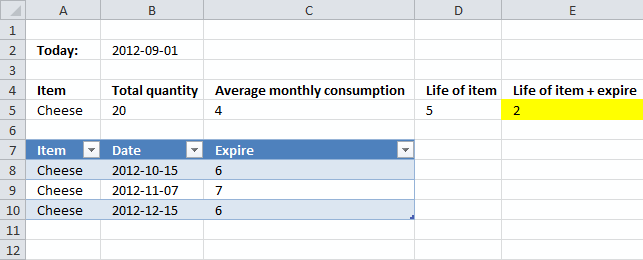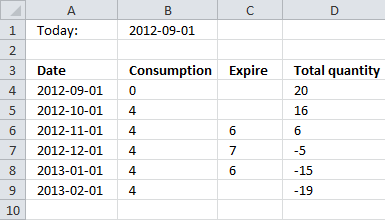Author: Oscar Cronquist Article last updated on October 12, 2012

The problem is with regards to analysing the remaining life of my inventory based on the quantity available and the monthly consumption. Its as follows :

Assuming today's date is 1st of September. I have 20 packets of cheese and the average monthly consumption is 4 packets. Ideally if the shelf life of those 20 packets is 6 months or more, then I can safely say that the total quantity will last for 5 months (20 divided by 4).However, with a slight twist in the data, assume that out of those 20 packets, 6 packets expire on 15 Oct, 7 packets expire on 10 Nov, and the remaining 6 on 15 December. Now I know that the total inventory is not gonna last for 5 months due to various expiry dates. If I calculate this manually, I come to know that the quantity will last for 3 and a half months.

How can i put all this in a formula to get the right answer ?

Thanks
HarounArray formula in cell E5:

=(YEAR((MAX(IF((((ROW(\$1:\$99)-1)*\$C\$5)+SUMIF(Table1[Date], "<="&DATE(YEAR(B2), MONTH(B2)+ROW(1:99)-1, DAY(B2)), Table1[Expire]))<B5, DATE(YEAR(B2), MONTH(B2)+ROW(1:99)-1, DAY(B2)), ""))))-YEAR(B2))*12+MONTH(MAX(IF((((ROW(\$1:\$99)-1)*\$C\$5)+SUMIF(Table1[Date], "<="&DATE(YEAR(B2), MONTH(B2)+ROW(1:99)-1, DAY(B2)), Table1[Expire]))<B5, DATE(YEAR(B2), MONTH(B2)+ROW(1:99)-1, DAY(B2)), "")))-MONTH(B2)

This formula returns months, rounded down. It can calculate up to 99 months. So how did the formula come up with the value 2? Take a look at this table.Today is 2012-09-01, a month later (2012-10-01) 4 items are consumed and 0 items have expired, total quantity 16.

2012-11-01 a total of 8 items are consumed and 6 items have expired, total quantity 6.

2012-12-01 a total of 12 items are consumed and 13 items have expired, total quantity -5.

All the inventory items are consumed/expired somewhere between 2012-11-01 and 2012-12-01.

2012-11-01 - 2012-09-01 = 2 months